# 4. Some examples.

Exempel 1: (Harmonisk oscillator.) Consider a mass m suspended in a spring with spring constant k>0. We release the mass from a starting point at time 0 and let it swing oscillate around an equilibrium point.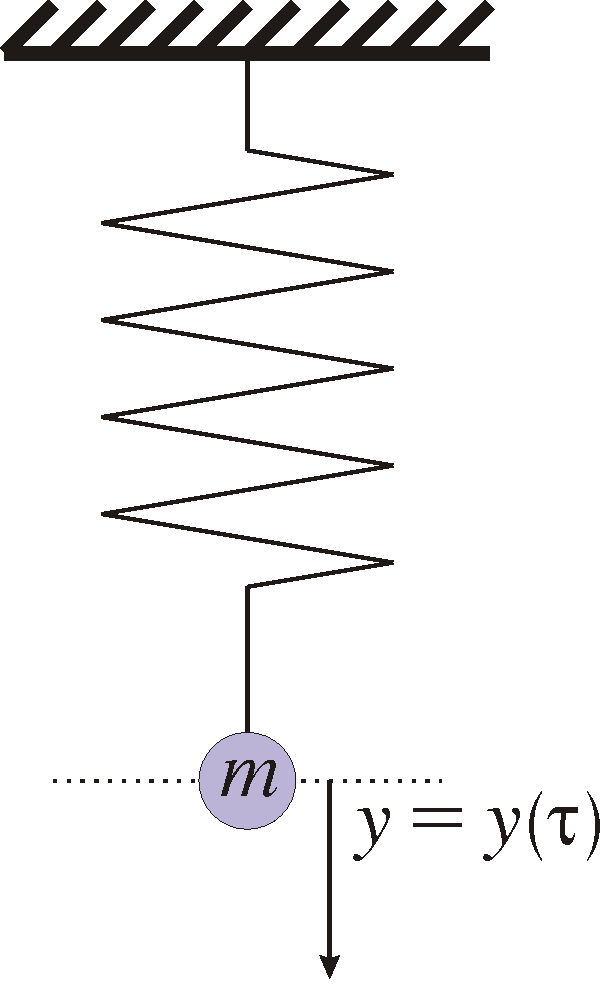If y=y(t) denotes the distance from the equilibrium point, then the kinetic energy is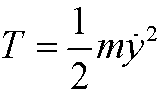and the potential energy isThe Lagrangian is thusand the action integralWe have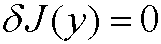when Lagrange’s equationis fulfilled, that is, whenThis is exactly Newton’s second law for the harmonic oscillator. Hamilton’s principle states that the motion will occur according to the above differential equation, with solutionFinally we note that the Hamiltonian isthe sum of the potential and kinetic energies, that is, the total energy.

Example 2: (Simple pendulum.) Consider a mass m suspended in a pendulum of length l. We denote the angle from the vertical line with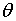and the arc length from the equilibrium point with sThe arc length s is connected to the angleby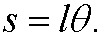Then we have the kinetic energyand the potential energy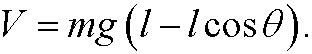Hamilton’s principle states that the motion will be such that the action integralhas a stationary value. Lagrange’s equation is in this caseThis implies that the equation of motion for the pendulum isThe Hamiltonian is in this case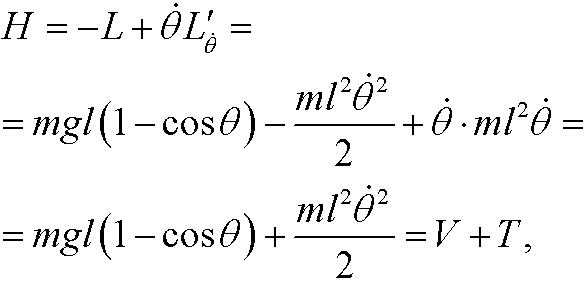that is, the total energy of the system.

Example 3: (Motion in a central force field.)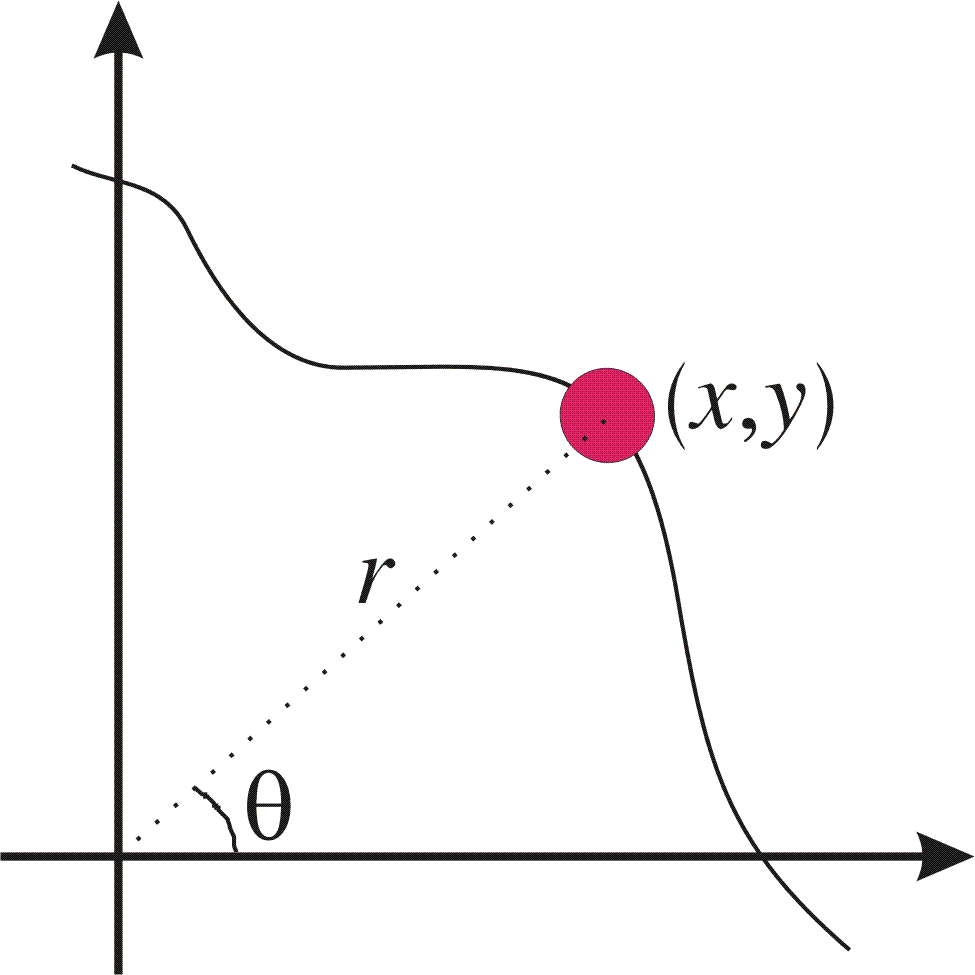Let us examine the motion in the plane of a mass m that is affected by a tensile force towards that is reciprocal to the square of the distance to origo, that isAs generalized coordinates we choose the polar coordinates r=r(t) and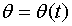. They are connected to the cartesian coordinates x and y by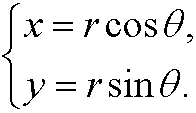This means that the kinetic energy for the system is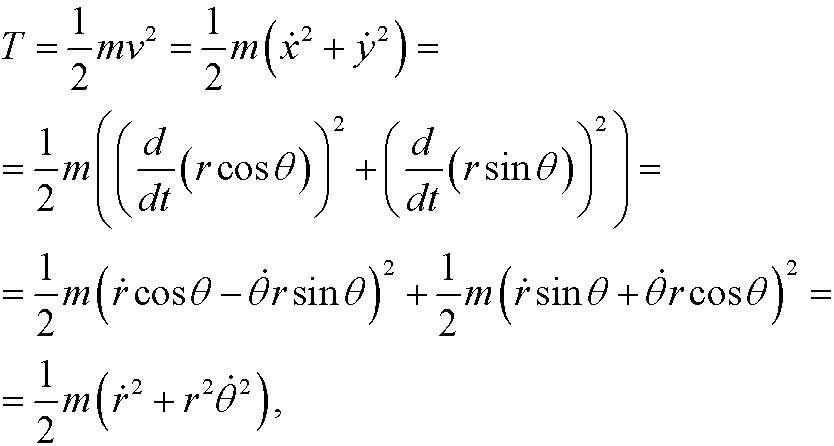and the potential energy is (up to an additive constant)(since the force is the negative gradient of the potential energy.) The Lagrangian is thusHamilton’s principle now states that we should findwhere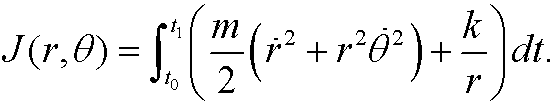In this case, Lagrange’s equations becomethat is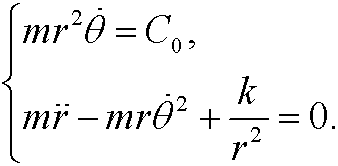This coupled system can be solved exactly (leading to Kepler’s laws), but it is out of the scope of this course.